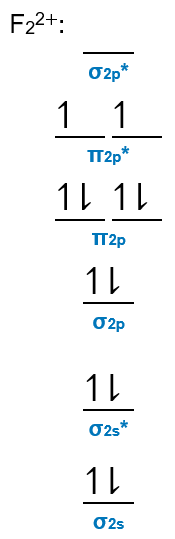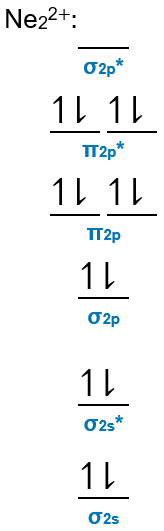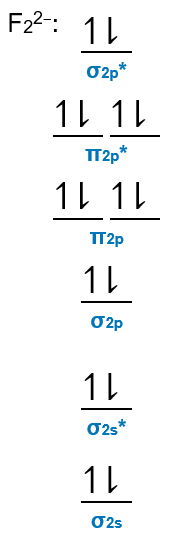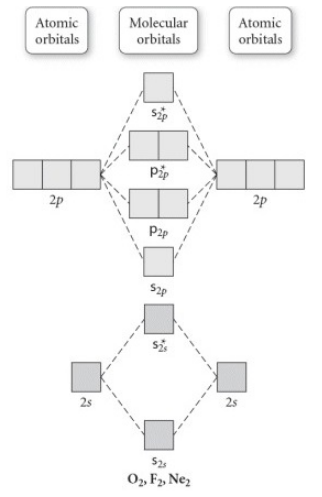# Problem: Use the molecular orbital diagram shown to determine which of the following is most stable.a. F22+b. Ne22+c. F22-d. O22+e. F2

###### FREE Expert Solution

Recall that the bond order tells us the strength and length of a bond: a higher bond order means the bond is stronger and shorter.

higher bond orderhigher bond strength → more stable

We can calculate for the bond order using:

bonding MOs → without an asterisk (e.g., σ1s)
antibonding MOs those with an asterisk (e.g., σ1s*)

a. F22+

Group            Valence Electrons
F        7A                   2 × 7 e14 e
From +2 charge:        – 2 e
Total:  12 valence eBond Order = 2.0

b. Ne22+

Group            Valence Electrons
Ne      8A                   2 × 8 e16 e
From +2 charge:        – 2 e
Total:  14 valence eBond Order = 1.0

c. F22-

Group            Valence Electrons
F        7A                   2 × 7 e14 e
From charge:        +2 e
Total:  16 valence e79% (479 ratings)###### Problem Details

Use the molecular orbital diagram shown to determine which of the following is most stable.

a. F22+

b. Ne22+

c. F22-

d. O22+

e. F2Frequently Asked Questions

What scientific concept do you need to know in order to solve this problem?

Our tutors have indicated that to solve this problem you will need to apply the MO Theory: Bond Order concept. You can view video lessons to learn MO Theory: Bond Order. Or if you need more MO Theory: Bond Order practice, you can also practice MO Theory: Bond Order practice problems.

What professor is this problem relevant for?

Based on our data, we think this problem is relevant for Professor Maxwell's class at UCF.